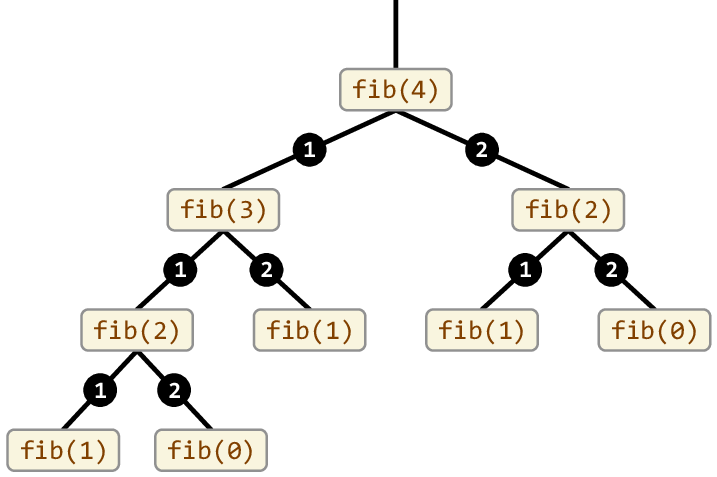##### DP #1: Introduction to Dynamic Programming

PREREQUISITES: BASIC UNDERSTANDING OF ALGORITHMS + UNDERSTANDING OF RECURSION

# What is dynamic programming?

Many problems can be solved by breaking them down into smaller problems, then taking these smaller problems and constructing the final solution. Usually, the solutions for these problems are implemented recursively - a function calls itself to solve the smaller subproblems, then eventually combines these solutions to form the answer for the larger problem. Let's take a look at a very simple example of recursive calculation with the fibonacci sequence. No doubt as you already know, the fibonacci sequence is defined as `f(n) = f(n - 1) + f(n - 2)` with `f(0) = 0` and `f(1) = 1`.

A simple recursive function to calculate the fibonacci sequence would look something like this:

``````function fib(n)
if (n == 0 or n == 1)
return 1
else
return fib(n - 1) + fib(n - 2)``````

The diagram below represents how this program would calculate the sequence:`Figure from https://www.researchgate.net/figure/Cilk-pseudocode-for-a-recursive-program-to-compute-Fibonacci-numbers-main_fig1_278965757`

To calculate the 4th fibonacci number, the program would have to calculate the 3rd and the 2nd. However, to calculate the 3rd fibonacci number, the program calculates the 2nd again! As you can see, with a recursive implementation, our program performs many duplicate calculations which will affect its speed.

Dynamic programming is an optimization to recursion that removes all of the inefficiencies of duplicate calculations. Instead of starting at the highest level problem and requesting solutions to smaller problems, dynamic programming starts at the smallest level problem, and uses the solutions to those problems to build up to the top level solutions. This is known as a bottom-up construction versus the top-down construction of recursion.

# What does dynamic programming look like?

Usually, dynamic programming is implemented iteratively with a table instead of recursively calling a function. The algorithm starts at the lowest levels of the problem, then iterates along and uses its previous solutions until it reaches the desired solution. A dynamic programming approach to calculate the fibonacci sequence would look something like this:

``````calculated = 0
x = 1
y = 1

while (calculated < desired)
y = x + y
x = y
calculated ++``````

This is one of the most simple examples of dynamic programming. In fact, you probably have already written this exact program before! The fibonacci sequence is especially easy to understand since we only require the previous solutions and the solution before that, so we can use 2 variables. However, in more complex dynamic programming problems, we usually store our previous solutions in a table so we can access them whenever we want.